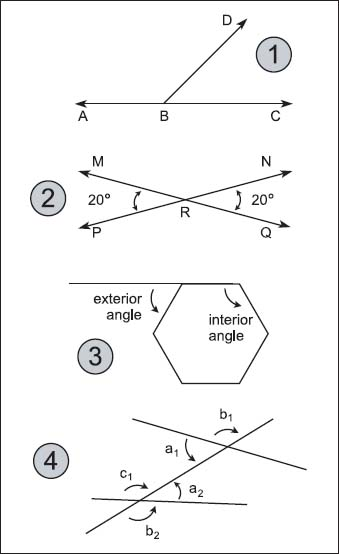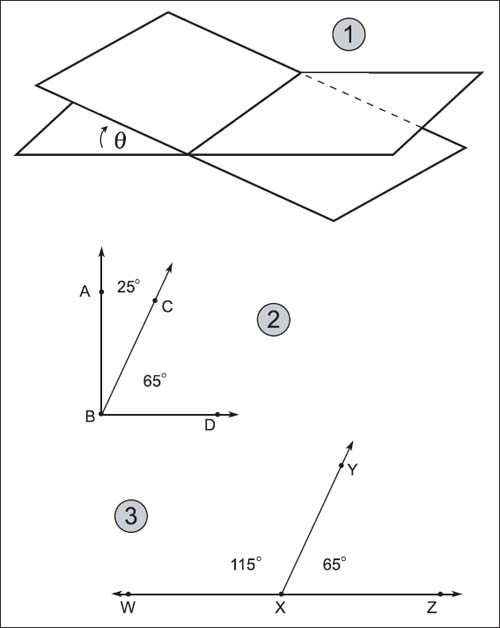# What are other types of angles?

There are numerous other types of angles, some of which are examined in the following list:

Adjacent angle—Adjacent angles are two angles side-by-side that have a common vertex (endpoint) and ray; angles are adjacent if a common line is between the other sides.

Vertical angles—Vertical angles are two angles whose sides form two pairs of opposite rays; because of this, vertical angles have no sides in common and are directly across from one another. They are also considered a pair of opposite congruent angles where two lines intersect. Vertical angles include intersecting lines that define a plane, with the vertical angles always in the same plane.

Congruent angles—Congruent angles have the same size and shape; vertical angles are considered to be congruent.

Exterior and interior angles—Interior angles are those inside a polygon, such as a triangle; exterior angles are outside the polygon formed by extending one ray (side) outside the polygon from a vertex.

Alternate and corresponding angles—A pair of angles that lie on opposite sides and opposite ends of a transversal (a line that cuts two or more lines in the same plane) are called alternate angles; both these angles are equal if the lines cut by the transversal are parallel. They are also broken into alternate interior angles (the “inside” angles) and alternate exterior angles (the “outside” angles). For two parallel lines, the alternate interior and exterior angles are equal—or congruent. Corresponding angles are pairs of angles that lie on the same sides and ends of a transversal.Among the types of angles are: 1. adjacent angles; 2. vertical (congruent) angles; and 3. exterior and interior angles. In illustration 4 we see alternate interior angles (a1 and a2), alternate exterior angles (b1 and b2), and corresponding angles (b1 and c1).

Dihedral angle—A dihedral (meaning “two bases”) angle is one of the four angles formed where two non-parallel planes meet.

Complementary angles—Two angles that add up to a right angle are complementary. For example, the two acute angles in a right triangle are always considered complementary, as their sum adds up to 90 degrees of the right triangle.

Supplementary angles—Two angles that add up to a straight angle (180 degrees) are supplementary. If the angles are both supplementary to the same angle, then the two angles are congruent.In the above, illustration 1 shows dihedral angle 6; illustration 2 shows complementary angles; and illustration 3 shows supplementary angles.

Close

This is a web preview of the "The Handy Math Answer Book" app. Many features only work on your mobile device. If you like what you see, we hope you will consider buying. Get the App